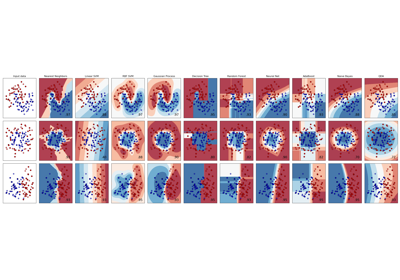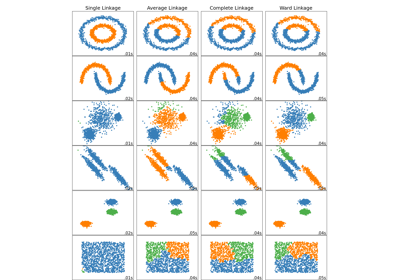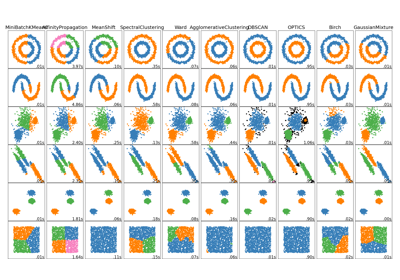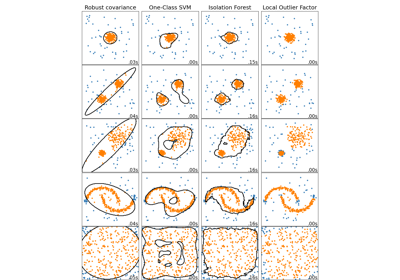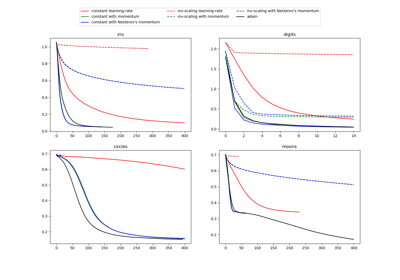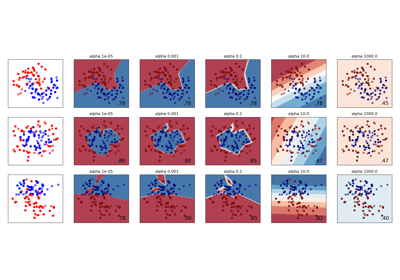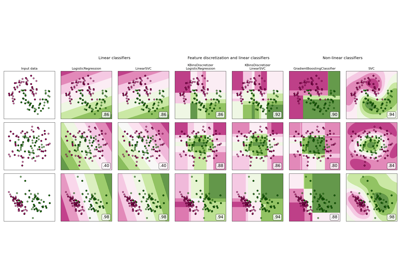# sklearn.datasets.make_moons¶

sklearn.datasets.make_moons(n_samples=100, *, shuffle=True, noise=None, random_state=None)[source]

Make two interleaving half circles

A simple toy dataset to visualize clustering and classification algorithms. Read more in the User Guide.

Parameters
n_samplesint or two-element tuple, optional (default=100)

If int, the total number of points generated. If two-element tuple, number of points in each of two moons.

shufflebool, optional (default=True)

Whether to shuffle the samples.

noisedouble or None (default=None)

Standard deviation of Gaussian noise added to the data.

random_stateint, RandomState instance, default=None

Determines random number generation for dataset shuffling and noise. Pass an int for reproducible output across multiple function calls. See Glossary.

Returns
Xarray of shape [n_samples, 2]

The generated samples.

yarray of shape [n_samples]

The integer labels (0 or 1) for class membership of each sample.

## Examples using sklearn.datasets.make_moons¶